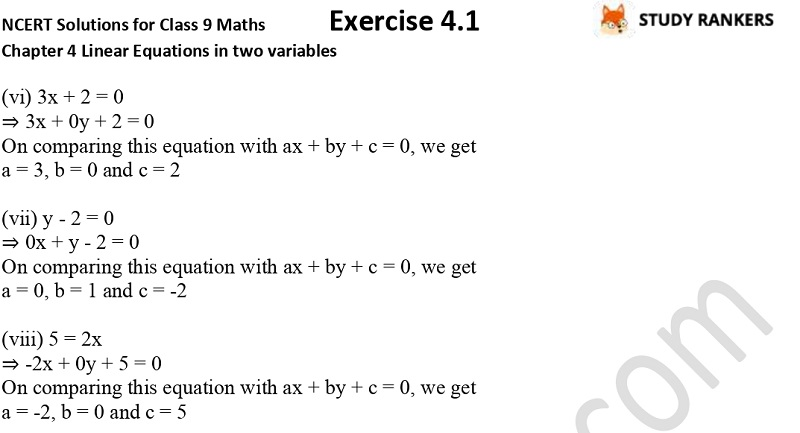## NCERT Solutions for Class 9 Maths Chapter 4 Linear Equations in Two Variables Exercise 4.1

Exercise 4.1 Chapter 4 Linear Equations in Two Variables Class 9 Maths NCERT Solutions is provided here that will be useful in knowing the fundamentals on the chapter. The formulas and points given will help you in higher classes so you must be well aware of the concepts. NCERT Solutions for Class 9 Maths prepared by subjects matter experts will provide you with the accurate and detailed solutions so you can always complete your homework on time. We have provided solutions to every difficult question so you can learn at ease.

There are only two questions in the Ex 4.1 in which you have to write a linear equation in two variables to represent given statement and express given linear equations in the form ax + by + c = 0.